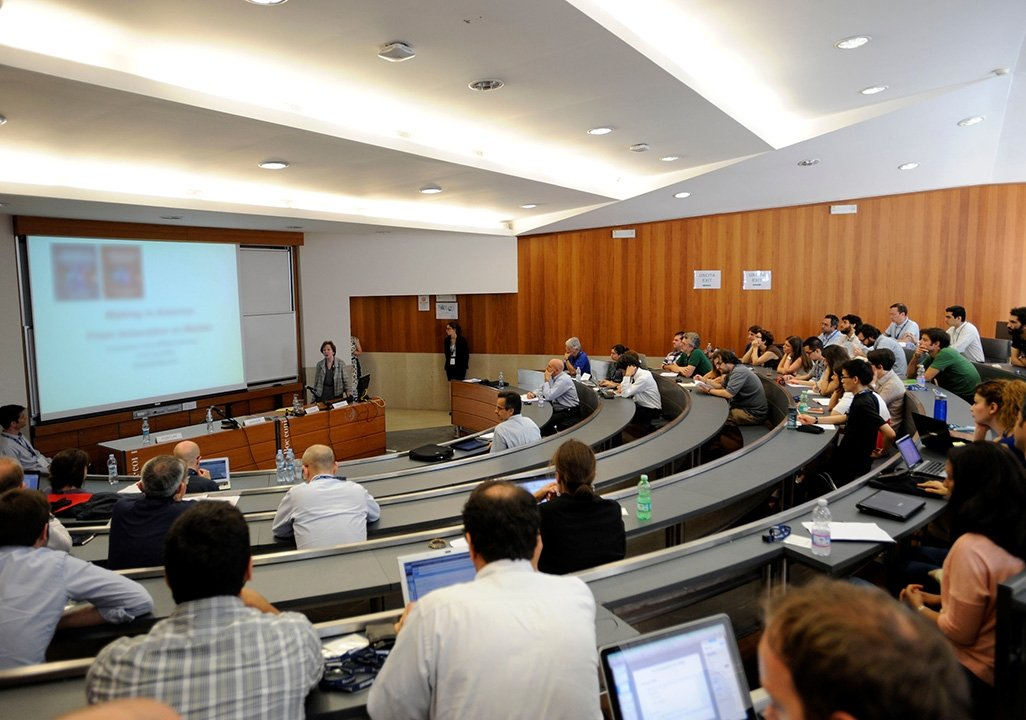## Daniela Tonon: Hamilton-Jacobi equations on infinite dimensional spaces corresponding to linearly controlled gradient flows of an energy functional

Seminars - Analysis and Applied Mathematics Seminar
Speakers
DANIELA TONON, Universita degli Studi di Padova
12:30 - 13:45
room 3-E4-SR03 (Rontgen)Abstract:

In this talk, we present a comparison principle for the Hamilton Jacobi (HJ) equation corresponding to a Mean Field control problem in which one linearly controls the gradient flow of an energy functional defined on a metric space. The main difficulties are given by the fact that the geometrical assumptions we require on the energy functional do not give any control on the growth of its gradient flow nor on its regularity. Therefore this framework is not covered by previous results on HJ equations on infinite dimensional spaces (whose study has been initiated in a series of papers by Crandall and Lions). Our proof of the comparison principle combines some rather classical ingredients, such as Ekeland’s perturbed optimization principle, with the use of the Tataru distance and of the regularizing properties of gradient flows in evolutional variational inequality formulation, that we exploit for constructing rigorous upper and lower bounds for the formal Hamiltonian.  Some hints on the existence of solutions will also be given.